# Tensor density

pseudo-tensor

A geometric object described in a coordinate system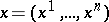by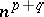components,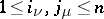, transforming under a change of coordinates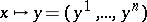according to the formula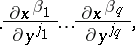where. The number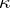is called the weight of the tensor density. When, the tensor density is a tensor (cf. Tensor on a vector space). Concepts such as type, valency, covariance, contravariance, etc. are introduced similar to the corresponding tensor concepts. Tensor densities of typesand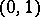are called vector densities. Tensor densities of type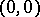are called scalar densities.

A tensor density as defined above is also called a relative tensor. One distinguishes between odd relative tensors of weight, which transform as above, and even relative tensors, which transform according to the same formula except thatis replaced by its absolute value. In [a2] an even tensor density is simply called a "tensor density" and an odd one is called a tensor-density.